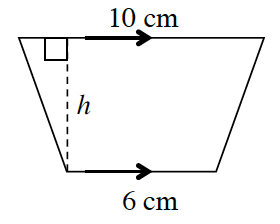### Home > CCG > Chapter 7 > Lesson 7.1.2 > Problem7-16

7-16.

The area of the trapezoid at right is $56\; \text{cm}^2$. What is $h$? Show all work.

What is the formula for the area of a trapezoid?
Use that to solve for $h$.

$\text{Height}=7$ cm